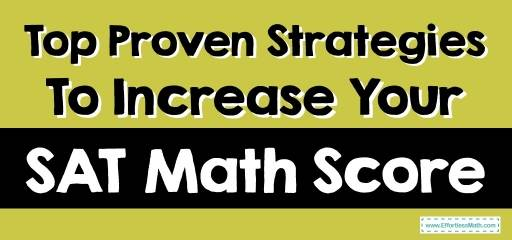# Top Proven Strategies To Increase Your SAT Math ScoreAre you dreading the math section of the SAT exam? Join the queue. You’re not the only one. Many students are pretty confident of their ability to ace their SAT exam, but the math section makes them feel queasy.

## Why You Should Have a High Math SAT Score?

If studying for the math section is something you’re doing too little of, then change. You need to make more effort because better math SAT scores better your chances of getting into better colleges, especially your dream college.

Some technical schools like Harvard, MIT, and Princeton are very critical in their selection process of admitting students.

So, if any of these schools is your choice, you have to do exceptionally well in the Math section and other quantitative and science subjects.

For students who have always had a tough time passing math, here are some proven strategies to help you perform exceptionally well in your SAT Math.

### Attempt all questions

Fortunately, you won’t be penalized for answering questions incorrectly in the new SAT, so you should try to attempt all questions since there’s the likelihood of a lucky guess.

The math section starts with easy questions, which should be a piece of cake for you, with constant practice.

Followed by medium questions, you should take your time answering these questions to answer all or most currently. Then you should spend your remaining time answering the difficult questions, these are usually tricky, but you can take your time since you’ve answered the previous questions.

The Absolute Best Book to Ace the SAT Math Test

### Impute the options

Since most of the math section is multiple-choice, it is definite that one of the options is the answer.

You can attribute the options into the equation one after the other to get the correct answer to make things easier. This strategy works best for percentages, algebraic equations, or questions that start with “in terms of.”

### Memorize math formulas

Math formulas are essential in answering math questions, and once you miss the formula, you likely miss the answer.

Geometry formulas are usually given in the questions, which is why you should focus on memorizing the other math formulas and practice math questions to test how versatile you are with the formulas.

### Discover your weak spots and work on them

Not only math but once you discover your weak spots, work on them, and spend more time practicing them than on other subjects you’re good at until you become a pro at them.

Try to understand why you keep getting those questions wrong. Once you know this, then you’ll know how to work on your weak points.

### Understand the math language

Most words in math language mean different than when used in the English language, which confuses most students.

An example of this is a sum, which means addition in math, or difference, which means distinguishing between two separate things, but in math means subtraction. Try to practice many word questions so you can be more familiar with these math languages.

### Time yourself while practicing questions

When sitting for the SATs, each question should be answered within 30 seconds, but if you haven’t answered enough practice questions, maximizing your time in the exam hall might prove difficult. So while practicing these questions, set a time limit for yourself.

If you spend extra time, try the questions ago until you can answer the questions within a specified time.

### Choose the right SAT practice questions.

When choosing your SAT practice materials, ensure not to choose outdated ones since SAT math sections get changed unexpectedly.

## Final Words

Even if you don’t like math, implementing these strategies will help you get better at Math, and you’re fast on your way to acing SAT math.

Best SAT Math Prep Resource

## More from Effortless Math for SAT Test …

### Are you looking for the best book for SAT math test?

Check out the Best Books for Students Preparing for the SAT Math Test.

### Need SAT Math Worksheets?

Check out SAT Math Worksheets: FREE & Printable.

### Here is a complete list of SAT Math Formulas.

The Best Books to Ace the SAT Math Test

## Have any questions about the SAT Test?

### What people say about "Top Proven Strategies To Increase Your SAT Math Score - Effortless Math: We Help Students Learn to LOVE Mathematics"?

No one replied yet.

X
49% OFF

Limited time only!

Save Over 49%

SAVE \$34

It was \$69.99 now it is \$35.99